Opalescent Aurora Color Melt
Last updated: September 26, 2017

# Opalescent Aurora Color Melt

ALL PHOTOS SLIDESHOW
GET THE HOW-TO• Formula A

Joico Blonde Life + 20-volume developer

• Formula B

Blonde Life + half 20-volume developer + half 30-volume developer

• Formula C

Blonde Life + 30-volume developer

• Formula D

2 parts LumiShine Demi-Permanent Liquid 9NV + 1 part LumiShine Demi-Permanent Liquid 10N + 3 drops LumiShine Demi-Permanent Liquid 1NV + 5-volume developer

• Formula E

Color Intensity True Blue

• Formula F

Color Intensity Kelly Green + Color Intensity Clear

• Formula G

Color Intensity Peach

• Formula H

Color Intensity Orchid# Opalescent Aurora Color Melt

Taking cues from the Northern Lights, this color melt extravaganza by Larisa Love (aka @larisadoll) uses two new hues from the Joico Color Intensity line: True Blue and Kelly Green. Check out how she takes fine weaves as accent pieces to create a dimensional melt, and watch the entire video here!

## COLOR FORMULAS• Formula A

Joico Blonde Life + 20-volume developer

• Formula B

Blonde Life + half 20-volume developer + half 30-volume developer

• Formula C

Blonde Life + 30-volume developer

• Formula D

2 parts LumiShine Demi-Permanent Liquid 9NV + 1 part LumiShine Demi-Permanent Liquid 10N + 3 drops LumiShine Demi-Permanent Liquid 1NV + 5-volume developer

• Formula E

Color Intensity True Blue

• Formula F

Color Intensity Kelly Green + Color Intensity Clear

• Formula G

Color Intensity Peach

• Formula H

Color Intensity Orchid

## HOW-TO STEPS•• Formula A

Joico Blonde Life + 20-volume developer

• Formula B

Blonde Life + half 20-volume developer + half 30-volume developer

• Formula C

Blonde Life + 30-volume developer

• Formula D

2 parts LumiShine Demi-Permanent Liquid 9NV + 1 part LumiShine Demi-Permanent Liquid 10N + 3 drops LumiShine Demi-Permanent Liquid 1NV + 5-volume developer

• Formula E

Color Intensity True Blue

• Formula F

Color Intensity Kelly Green + Color Intensity Clear

• Formula G

Color Intensity Peach

• Formula H

Color Intensity Orchid

1

Prelighten the hair using Formulas A, B and C depending on where you are working on the head. Pre-tone with Formula D, processing for 5 to 20 minutes until desired outcome is achieved.

•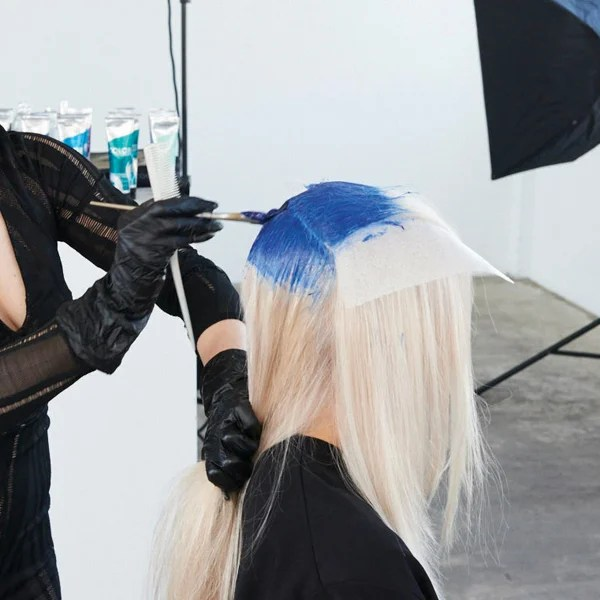• Formula A

Joico Blonde Life + 20-volume developer

• Formula B

Blonde Life + half 20-volume developer + half 30-volume developer

• Formula C

Blonde Life + 30-volume developer

• Formula D

2 parts LumiShine Demi-Permanent Liquid 9NV + 1 part LumiShine Demi-Permanent Liquid 10N + 3 drops LumiShine Demi-Permanent Liquid 1NV + 5-volume developer

• Formula E

Color Intensity True Blue

• Formula F

Color Intensity Kelly Green + Color Intensity Clear

• Formula G

Color Intensity Peach

• Formula H

Color Intensity Orchid

2

Begin by parting the hair into three sections: two on either side of the center part from the back of the ear forward, and one section in the back. Apply Formula E at the root in a teardrop shape.

•• Formula A

Joico Blonde Life + 20-volume developer

• Formula B

Blonde Life + half 20-volume developer + half 30-volume developer

• Formula C

Blonde Life + 30-volume developer

• Formula D

2 parts LumiShine Demi-Permanent Liquid 9NV + 1 part LumiShine Demi-Permanent Liquid 10N + 3 drops LumiShine Demi-Permanent Liquid 1NV + 5-volume developer

• Formula E

Color Intensity True Blue

• Formula F

Color Intensity Kelly Green + Color Intensity Clear

• Formula G

Color Intensity Peach

• Formula H

Color Intensity Orchid

3

Continue to apply the shadow root in the back section, bringing the color down about 3 to 4 inches.

•• Formula A

Joico Blonde Life + 20-volume developer

• Formula B

Blonde Life + half 20-volume developer + half 30-volume developer

• Formula C

Blonde Life + 30-volume developer

• Formula D

2 parts LumiShine Demi-Permanent Liquid 9NV + 1 part LumiShine Demi-Permanent Liquid 10N + 3 drops LumiShine Demi-Permanent Liquid 1NV + 5-volume developer

• Formula E

Color Intensity True Blue

• Formula F

Color Intensity Kelly Green + Color Intensity Clear

• Formula G

Color Intensity Peach

• Formula H

Color Intensity Orchid

4

After the shadow root is applied in the back section, take the entire back section and part it with a large zigzag parting from ear to ear. Then, take a fine weave from the zigzag and clip it out of the way.

•• Formula A

Joico Blonde Life + 20-volume developer

• Formula B

Blonde Life + half 20-volume developer + half 30-volume developer

• Formula C

Blonde Life + 30-volume developer

• Formula D

2 parts LumiShine Demi-Permanent Liquid 9NV + 1 part LumiShine Demi-Permanent Liquid 10N + 3 drops LumiShine Demi-Permanent Liquid 1NV + 5-volume developer

• Formula E

Color Intensity True Blue

• Formula F

Color Intensity Kelly Green + Color Intensity Clear

• Formula G

Color Intensity Peach

• Formula H

Color Intensity Orchid

5

On the bottom section, melt in Formula F, using your fingers in a scissor motion to blend the colors together.

•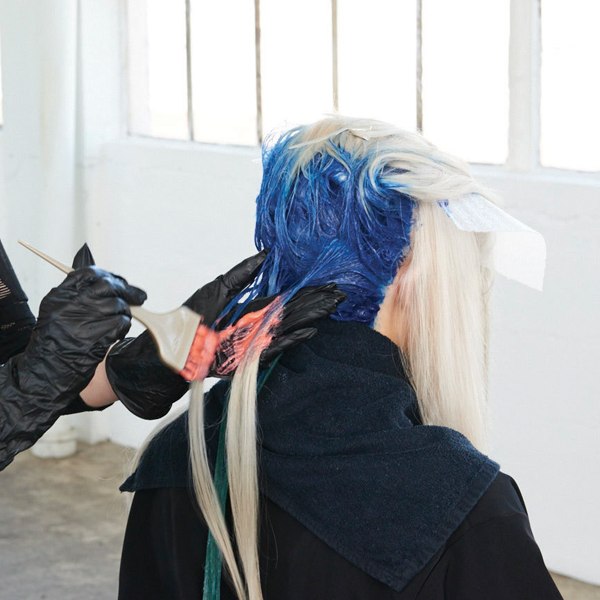• Formula A

Joico Blonde Life + 20-volume developer

• Formula B

Blonde Life + half 20-volume developer + half 30-volume developer

• Formula C

Blonde Life + 30-volume developer

• Formula D

2 parts LumiShine Demi-Permanent Liquid 9NV + 1 part LumiShine Demi-Permanent Liquid 10N + 3 drops LumiShine Demi-Permanent Liquid 1NV + 5-volume developer

• Formula E

Color Intensity True Blue

• Formula F

Color Intensity Kelly Green + Color Intensity Clear

• Formula G

Color Intensity Peach

• Formula H

Color Intensity Orchid

6

Take the weaved-out section and melt Formula G in through the Formula E. This is the accent section. Apply meche between colors so they don’t bleed.

Pro Tip: Combing is important to make sure color is saturated.

•• Formula A

Joico Blonde Life + 20-volume developer

• Formula B

Blonde Life + half 20-volume developer + half 30-volume developer

• Formula C

Blonde Life + 30-volume developer

• Formula D

2 parts LumiShine Demi-Permanent Liquid 9NV + 1 part LumiShine Demi-Permanent Liquid 10N + 3 drops LumiShine Demi-Permanent Liquid 1NV + 5-volume developer

• Formula E

Color Intensity True Blue

• Formula F

Color Intensity Kelly Green + Color Intensity Clear

• Formula G

Color Intensity Peach

• Formula H

Color Intensity Orchid

7

Repeat the process, using Formula H instead of Formula G as the accent color. Continue up the back, alternating Formulas G and F as the accent color on the small weaved-out sections.

•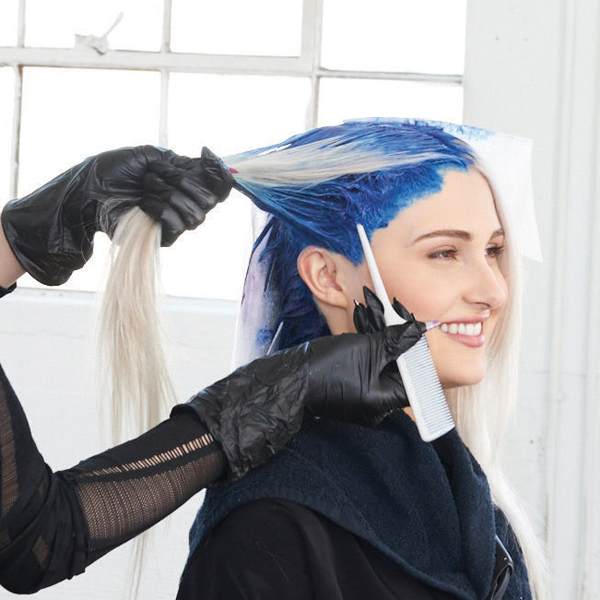• Formula A

Joico Blonde Life + 20-volume developer

• Formula B

Blonde Life + half 20-volume developer + half 30-volume developer

• Formula C

Blonde Life + 30-volume developer

• Formula D

2 parts LumiShine Demi-Permanent Liquid 9NV + 1 part LumiShine Demi-Permanent Liquid 10N + 3 drops LumiShine Demi-Permanent Liquid 1NV + 5-volume developer

• Formula E

Color Intensity True Blue

• Formula F

Color Intensity Kelly Green + Color Intensity Clear

• Formula G

Color Intensity Peach

• Formula H

Color Intensity Orchid

8

Move to the right front section. Apply the shadow root with Formula E, keeping the teardrop shape in mind and bringing color about 1 ½-2 inches from scalp. Take a diagonal-forward slice from the temple to the top of the crown. Weave a section from the slice, with the weave thicker in the front and thinner toward the back. Clip the weave section of the way.

Pro Tip: Keeping the weave thicker in front and thinner toward the back gives a money piece in the front for a pop and helps blend with the back section.

•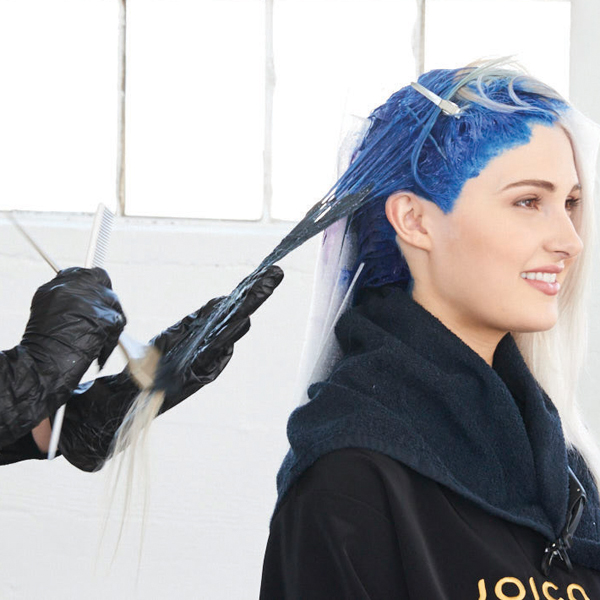• Formula A

Joico Blonde Life + 20-volume developer

• Formula B

Blonde Life + half 20-volume developer + half 30-volume developer

• Formula C

Blonde Life + 30-volume developer

• Formula D

2 parts LumiShine Demi-Permanent Liquid 9NV + 1 part LumiShine Demi-Permanent Liquid 10N + 3 drops LumiShine Demi-Permanent Liquid 1NV + 5-volume developer

• Formula E

Color Intensity True Blue

• Formula F

Color Intensity Kelly Green + Color Intensity Clear

• Formula G

Color Intensity Peach

• Formula H

Color Intensity Orchid

9

Melt Formula F into the shadow root on bottom section.

•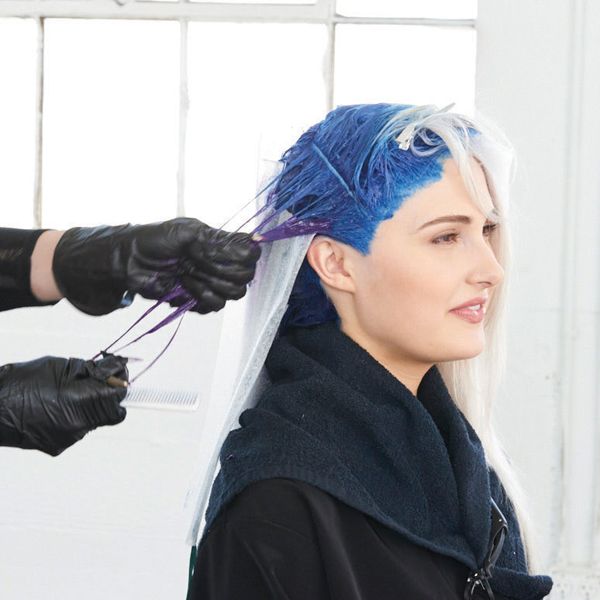• Formula A

Joico Blonde Life + 20-volume developer

• Formula B

Blonde Life + half 20-volume developer + half 30-volume developer

• Formula C

Blonde Life + 30-volume developer

• Formula D

2 parts LumiShine Demi-Permanent Liquid 9NV + 1 part LumiShine Demi-Permanent Liquid 10N + 3 drops LumiShine Demi-Permanent Liquid 1NV + 5-volume developer

• Formula E

Color Intensity True Blue

• Formula F

Color Intensity Kelly Green + Color Intensity Clear

• Formula G

Color Intensity Peach

• Formula H

Color Intensity Orchid

10

Use Formula H on the smaller weave section. Repeat up the head, alternating the accent section colors.

•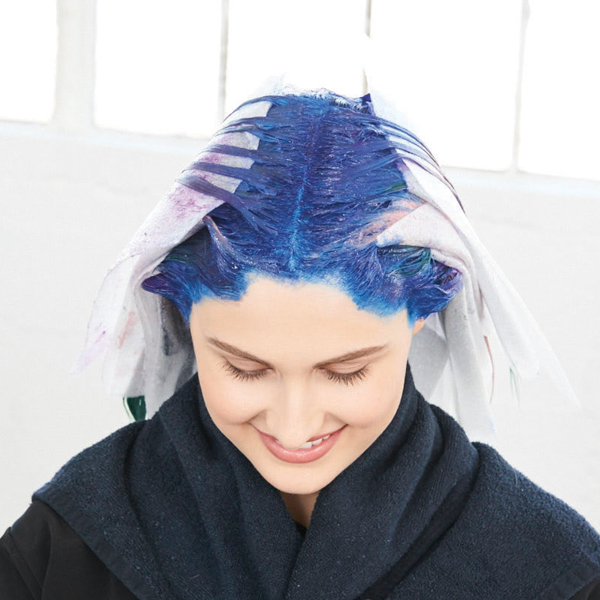• Formula A

Joico Blonde Life + 20-volume developer

• Formula B

Blonde Life + half 20-volume developer + half 30-volume developer

• Formula C

Blonde Life + 30-volume developer

• Formula D

2 parts LumiShine Demi-Permanent Liquid 9NV + 1 part LumiShine Demi-Permanent Liquid 10N + 3 drops LumiShine Demi-Permanent Liquid 1NV + 5-volume developer

• Formula E

Color Intensity True Blue

• Formula F

Color Intensity Kelly Green + Color Intensity Clear

• Formula G

Color Intensity Peach

• Formula H

Color Intensity Orchid

11

Repeat the entire process on the other side.

•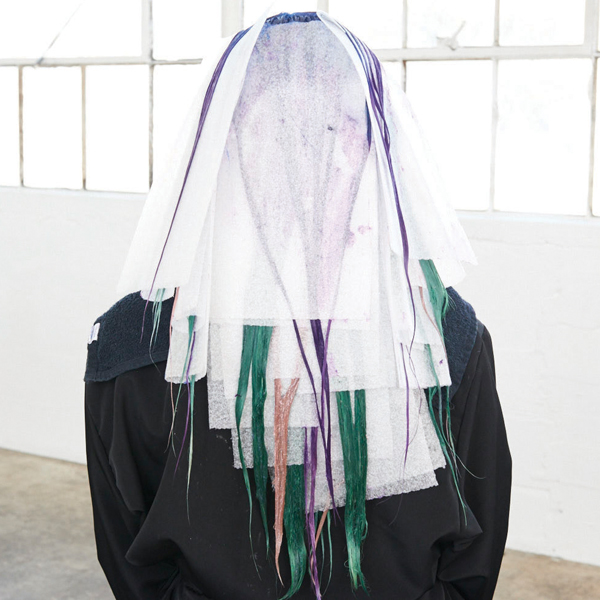• Formula A

Joico Blonde Life + 20-volume developer

• Formula B

Blonde Life + half 20-volume developer + half 30-volume developer

• Formula C

Blonde Life + 30-volume developer

• Formula D

2 parts LumiShine Demi-Permanent Liquid 9NV + 1 part LumiShine Demi-Permanent Liquid 10N + 3 drops LumiShine Demi-Permanent Liquid 1NV + 5-volume developer

• Formula E

Color Intensity True Blue

• Formula F

Color Intensity Kelly Green + Color Intensity Clear

• Formula G

Color Intensity Peach

• Formula H

Color Intensity Orchid

12

Process for 25 min, shampoo with Joico Co+Wash Color Whipped Cleansing Conditioner.

•• Formula A

Joico Blonde Life + 20-volume developer

• Formula B

Blonde Life + half 20-volume developer + half 30-volume developer

• Formula C

Blonde Life + 30-volume developer

• Formula D

2 parts LumiShine Demi-Permanent Liquid 9NV + 1 part LumiShine Demi-Permanent Liquid 10N + 3 drops LumiShine Demi-Permanent Liquid 1NV + 5-volume developer

• Formula E

Color Intensity True Blue

• Formula F

Color Intensity Kelly Green + Color Intensity Clear

• Formula G

Color Intensity Peach

• Formula H

Color Intensity Orchid

13

Finished look.